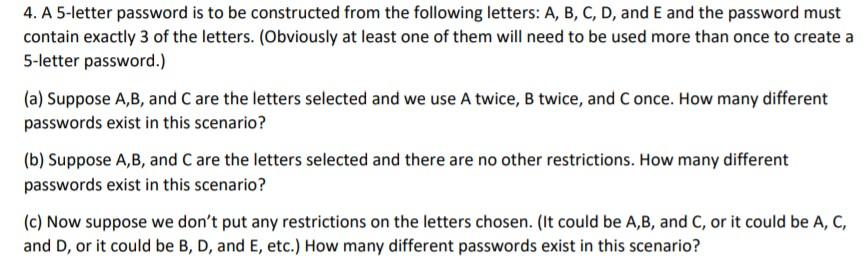# Question Solved1 Answer4. A 5-letter password is to be constructed from the following letters: A, B, C, D, and E and the password must contain exactly 3 of the letters. (Obviously at least one of them will need to be used more than once to create a 5-letter password.) (a) Suppose A,B, and C are the letters selected and we use A twice, B twice, and Conce. How many different passwords exist in this scenario? (b) Suppose A,B, and C are the letters selected and there are no other restrictions. How many different passwords exist in this scenario? (c) Now suppose we don't put any restrictions on the letters chosen. (It could be A,B, and C, or it could be A, C, and D, or it could be B, D, and E, etc.) How many different passwords exist in this scenario?H8P7M5 The Asker · Probability and StatisticsTranscribed Image Text: 4. A 5-letter password is to be constructed from the following letters: A, B, C, D, and E and the password must contain exactly 3 of the letters. (Obviously at least one of them will need to be used more than once to create a 5-letter password.) (a) Suppose A,B, and C are the letters selected and we use A twice, B twice, and Conce. How many different passwords exist in this scenario? (b) Suppose A,B, and C are the letters selected and there are no other restrictions. How many different passwords exist in this scenario? (c) Now suppose we don't put any restrictions on the letters chosen. (It could be A,B, and C, or it could be A, C, and D, or it could be B, D, and E, etc.) How many different passwords exist in this scenario?
More
Transcribed Image Text: 4. A 5-letter password is to be constructed from the following letters: A, B, C, D, and E and the password must contain exactly 3 of the letters. (Obviously at least one of them will need to be used more than once to create a 5-letter password.) (a) Suppose A,B, and C are the letters selected and we use A twice, B twice, and Conce. How many different passwords exist in this scenario? (b) Suppose A,B, and C are the letters selected and there are no other restrictions. How many different passwords exist in this scenario? (c) Now suppose we don't put any restrictions on the letters chosen. (It could be A,B, and C, or it could be A, C, and D, or it could be B, D, and E, etc.) How many different passwords exist in this scenario?